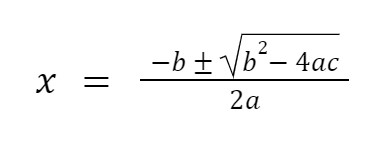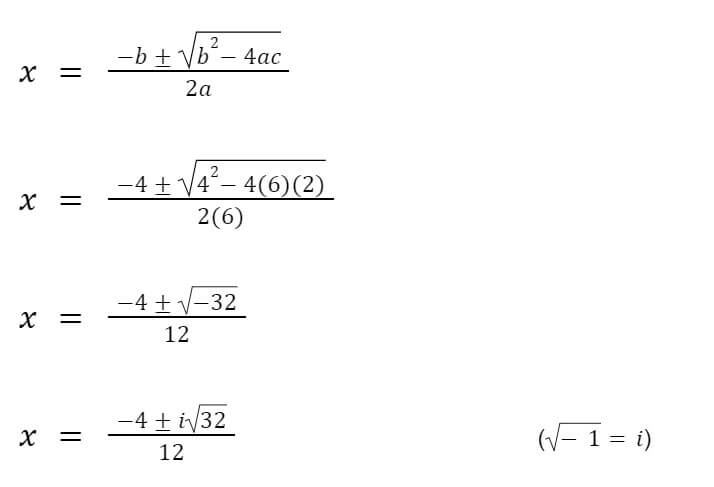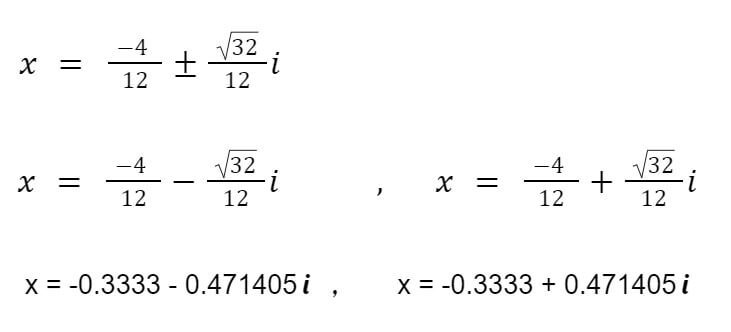Enter the coefficients of x in the quadratic formula and equation calculator to find its roots.

ax² + bx + c = 0This advanced quadratic equation and formula solver computes the roots of a quadratic equation. It also gives the detail of the whole process along with a plotted graph.

## What is a Quadratic equation?

“ A polynomial expression which has the maximum degree of variable as 2.”

A quadratic equation is of the form ax+ bx + c = 0. Where a, b, and c are the coefficients and are known. While variables like “x” are unknown.

To find the values of X, roots, we use a formula known as the quadratic formula:Where (b2 - 4ac) is known as a discriminant.

## Nature of the roots

The value of the discriminant determines the nature of the roots whether they are real or imaginary (complex) roots. Here is a guide to learn it beforehand:

• If discriminant > 0 , roots are real
• If discriminant = 0, one real root
• If discriminant < 0, imaginary roots

## How to solve Quadratic equations?

Example:

Solve 6x2 + 4x + 2 = 0.

Solution:

Step 1: Identify the Coefficients.

a = 6, b = 4 and c = 2

Step 2: Use the quadratic formula.So, the roots are complex. These radicals can be further solved as